Next: Practical considerations Up: Tools for time series Previous: Tools for time series   Contents

### Reconstructing the state-space

The first problem in modelling a time series like the one described by Equations (2.6) and (2.7) is to try to reconstruct the original state-space or its equivalent, i.e. to find the structure of the manifold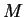.

Two spaces are topologically equivalent if there exists a continuous mapping with a continuous inverse between them. In this case it is sufficient that the equivalent structure is a part of a larger entity, the rest can easily be ignored. Thus the interesting concept is embedding, which is defined as follows.

Definition 1   A function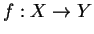is an embedding if it is a continuous mapping with a continuous inverse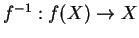from its range to its domain.

Whitney showed in 1936  that any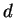-dimensional manifold can be embedded into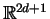. The theorem can be extended to show that with a proper definition of almost all for an infinite-dimensional function space, almost all smooth mappings from given-dimensional manifold toare embeddings .

Having only a single time series produced by Equation (2.7), how does one get those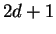different coordinates? This problem can usually be solved by introducing so called delay coordinates .

Definition 2   Let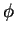be a flow on a manifold,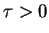, and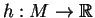a smooth measurement function. The delay coordinate map with embedding delay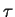,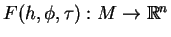is defined by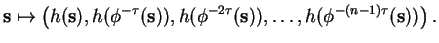Takens proved in 1980  that such mappings can indeed be used to reconstruct the state-space of the original dynamical system. This result is known as Takens' embedding theorem.

Theorem 3 (Takens)   Letbe a compact manifold of dimension. For triples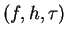, where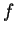is a smooth vector field onwith flow,a smooth measurement function and the embedding delay, it is a generic property that the delay coordinate map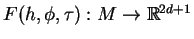is an embedding.

Takens' theorem states that in the general case, the dynamics of the system recovered by delay coordinate embedding are the same as the dynamics of the original system. The exact mathematical definition of this in general'' is, however, somewhat more complicated .

Definition 4   A subset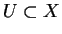of a topological space is residual if it contains a countable intersection of open dense subsets. A property is called generic if it holds in a residual set.

According to Baire's theorem, a residual set cannot be empty. Unfortunately that is about all that can be said about it. Even in Euclidean spaces, a residual set can be of arbitrarily small measure. With a proper definition for almost all in infinite-dimensional spaces and slightly different assumptions, Sauer et al. showed in 1991 that the claim of Takens' theorem actually applies almost always .Next: Practical considerations Up: Tools for time series Previous: Tools for time series   Contents
Antti Honkela 2001-05-30